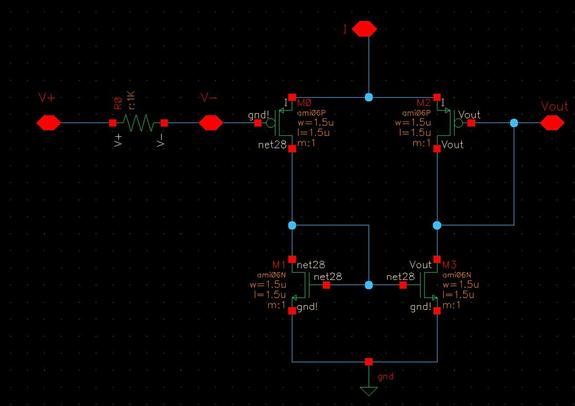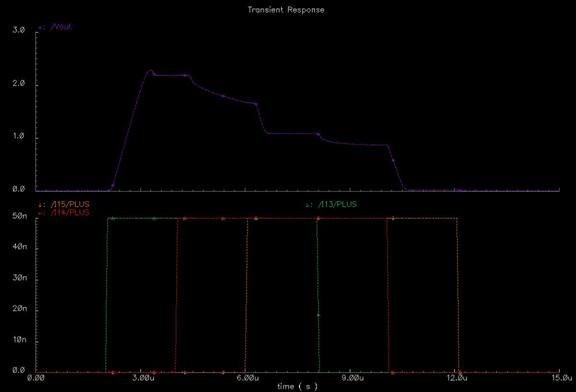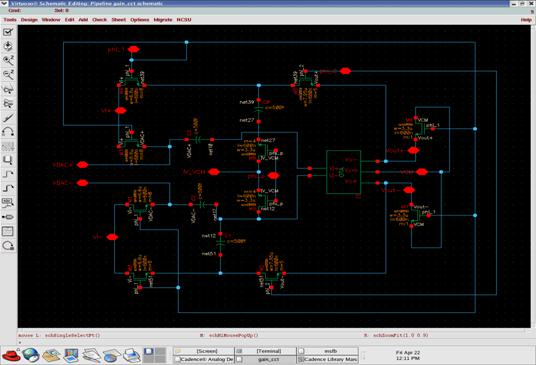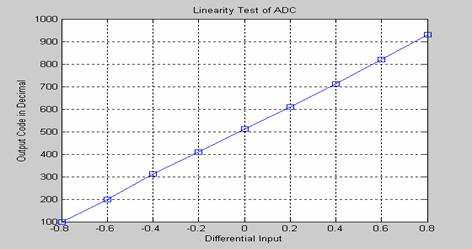Spring 2005

3-D Depth Finding Imager with Digital Readout

Ndubuisi Ekekwe, Yehuda Graber and Andy Barnes

(nekekwe1 (at) jhu.edu), (ygraber1 (at) jhu.edu); (rancore1 (at) hotmail.com)# Project Overview

The objective of the project is the design of an imager chip for laser-scanned 3-D depth/range finding. The project is divided into two modules: Pixel Array/Centroid Detection and Analog-to-Digital Conversion (ADC). The pixel array/centroid detector tracks the centroid of the laser beam as its scans the object while the ADC converts the analog output of the centroid into digital format. The conversion into digital ensures that the result is readily available for further processing in the digital domain. The pixel array/centroid detector is described first.

# Pixel Array/Centroid Detection

The pixel and centroid system works in the following way. A laser is scanned across an object continuously in the vertical direction and discretely in the horizontal direction. The laser beam reflects off the surface of the target. Because the imager observes the object from a different angle than that of the laser source, the surface depth is recorded as a horizontal deflection of the projected laser beam. The figure below illustrates the system overview.As the laser illuminates more than a single pixel, a distribution of the incident light intensity is recorded on the imager. The discrete distribution is observed as photocurrents along columns of pixels of the imager. If we were to just use a winner-takes-all decision circuit to extract the horizontal deflection of the laser beam, we would be getting single pixel accuracy. By finding the center of this distribution we find our deflection value with sub-pixel accuracy.Only deflections in one axis are recorded and used. Therefore there is no need for a full N x N array of photo pixels. At the same time, the decision circuit will require that all the pixel output currents in a single column be summed together. Rather than summing the pixel outputs, a single large photo column can be used.  This has the added benefit of increasing the amount of photosensitive area that can be squeezed into the same space.

The original goal was to achieve 30 fps at an accuracy of 128 x 128 pixels. Each photo column should have the same area as 1 column of square photo pixels.  This large photosensitive area has a high capacitance. Therefore speed was the main design objective of the photo pixel support circuitry. Photodiodes were used rather than phototransistors for two reasons. The first is that phototransistors have a floating base and therefore react slower. Secondly photodiodes can be made from a junction of N-well and P-substrate, with significantly lower capacitance than a direct N-active to P-substrate junction.At 128 x 128 pixel accuracy the photodiode has a capacitance of around 100 fF. This is large enough to hinder a 30 fps rate. Therefore the following pixel support circuitry was suggested. See schematic above. The output of the photodiode goes into a cascoded inverting amplifier which is then fed back to the output via an NMOS. This feedback circuit clamps the voltage on the N-Well. By keeping the voltage close to a constant, the photodiode does not need to charge and discharge the capacitance every time the photocurrent changes. Therefore the desired speed is achieved, as shown in the simulation below.An interesting side benefit of clamping the voltages is the following. Normally a minimum of 18 lambda spacing is required between N-Wells at different potentials. But since the potential on the photodiode is being clamped any two adjacent photodiodes will be at very similar voltages. Therefore we can resort to the minimum N-Well spacing distance of 6 lambda. It should be mentioned that there is little side effect to photocurrent leakage from one photo pixel to the next, as this should not affect the centroid of the distribution to first order. Below is the layout of the photopixel support circuitry (left) and the centroid (right).The next stage is the decision circuit. The centroid circuit can be seen in the schematic below . The centroid circuit is a transconductance amplifier that has its tail current supplied by the previous stage. Each element of the centroid circuit implements a variable transconductance.The individual elements of the centroid calculator are strung together with a string of resistors, and a variable transconductance tapped off of the line after each resistor. Therefore each centroid element has an input at equally spaced voltages.  A sample setup can be viewed in the following schematic.The centroid calculator attempts to calculate:   Σ I(xi)xi / ΣI(xi). It is a well known that the transconductance of a differential pair is only linear very close to the origin. As the differential input increases, the transconductance saturates. This has significant implications for the centroid calculation. For those elements of the centroid circuit which are close in proximity to the peak of the distribution, they will be averaged together weighted by the input photocurrents. The statistical mean will be calculated. But those on the edges of the distribution will not be weighted, just counted. Here the circuit is calculating the statistical median.The example distribution above shows why this is quite a benefit. Looking at the peak of the distribution, we want to find the mean. But if you notice the secondary peak, we do not want those values to influence the centroid so that it is in between the two peaks. We do not want the value of the secondary peak to influence the placement of the centroid. We only want the existence and placement of the secondary peak to influence the centroid.

Below is a simulation of the centroid test schematic. The output of the centroid is converted into digital form by the ADC which is described next.Analog-to-Digital Converter

For transmission of data at the video rate speed, a fast analog-to-digital converter (ADC) is required. Among different ADC types, the Pipelined ADC offered the best choice. It offers sampling rate up to 100MS/s, high resolution, low power consumption with good dynamic performance suitable for interface with the centroid and pixel array modules. In addition, it has a complexity of N (number of bits) making it a good choice in CMOS imager with constraint on die area.

The ADC uses pipeline 1.5 bit/stage architecture with nine stages, as shown I below. Each stage resolves two bits with a sub-ADC, subtracts this value from its input, and amplifies the resulting residue by a gain of two [1, 2]. The last stage of the ADC does not have the gain stage; rather the two bits are digitally corrected as the two LSBs of the ADC. The resulting 18 bits from the nine stages are combined with digital error correction after passing through shift registers to yield 10 bits at the output of the ADC. The shift is used to store the digital bits of the earlier stages until the N-1 stage bit is obtained.The major macros in the ADC are briefly discussed below:

The sub-ADC quantizes the input signal and provides the intermediate bits for each stage. For 1.5 bits per stage architecture sub-ADC can have one of three binary states as an output: 00, 01, 10. The sub-ADC consists of two differential comparators with thresholds set at +VR/4  and _VR/4  , where +VR and _VR represent the range of the differential input signal. It consists of two differential comparators. These outputs are sent to sub-DAC block.# Sub-DAC

The sub-DAC supplies the gain stage with the analog voltage level that represents the quantized portion of the input sample. The quantized portion is subtracted from the input signal to create a residue, which is amplified by 2 and sent to the next stage. In this design, the sub-DACA also generates the two bits that are sent to the shift register and error correction logic to obtain the bit for each of the stages. This stage acts like an analog MUX using the circuitry to choice  outputs of _VR/2 , 0, +VR/2 based on different input bits.# Amplifier Design

Due to simplicity ( ie die area) and need for high speed of operation in the imager, a telescopic operational trans-conductance amplifier was used. It uses a wide swing bias network combined with a common mode feedback and gain boosting to deliver good gain and BW necessary for our design. The input currents are mirrored with a cascaded configuration to produce the output currents. The telescopic architecture puts both the input differential pair and the output on the same two current branches. This approach eliminates the noise problems caused by the current mirrors and also leads to a more direct signal path, which allows for higher speed.Gain Circuit

This stage is used to obtain the residue by subtracting the sub-DAC output from the original input and route it to the next stage. It contains a S/H circuit, an OTA, integrating capacitors and switches. The output of the sub-DAC and Vref are stored in the capacitors which using the differential pair of the OTA makes comparison to generate the residue. This value is then amplified in the amplifier circuitry and sent to the next stage of the pipeline ADC.# Clock Design

The clock is a typical two-phase non-overlapping clock. To eliminate switch dependent charge injection, the two phases have two pulses with slightly different duty cycles.

# Error correction Logic

Since the design is pipelined, all the bits are not ready at the same time. A shift register is used to hold the earlier bits until the last bit of the ADC is obtained. An adder system is used to implement the error correction. It basically involves the use of outputs bits of the shift register as inputs into the adder.

# Simulation Results

A linearity performance was performed on the ADC. The result of the simulation was processed with Matlab and  is presented below.The table below shows the summary of the test result of the ADC at 27oC.

 Technology AMI C5N Resolution 10bits Conversion rate 50MS/s Input range +/- 0.8V(Vcm =2.5V) Power dissipation 87.78mW Latency 6 initial cycles Vdd 3.3V

References

        Andrew M. Abo and Paul R. Gray, “A 1.5-V, 10-bit,14.3-MS/s CMOS Pipeline Analog-to-Digital Converter”, IEEE J. Solid-State Circuits, vol.34, pp. 599-605, May 1999.

        Analog Integrated Circuit Design, D. J. Johns and K. Martin, Wiley, 1997.

        Alma Delic-Ibukic, “10-bit, 50MHz, pipeline A/D Converter”, Jan 13, 2004 {online: www.eece.maine.edu}

        Cohen, Marc, Cauwenberghs, Gert and Vorontsov, Mikhail A., “Image Sharpness and Beam Focus VLSI Sensor for Adaptive Optics,” IEEE Sensors Journal, Vol 2, No. 6, December 2003.